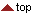## Performance Evaluation

For each database and for each algorithm:

• Each sample in the subset A is matched against the remaining samples of the same finger to compute the False Non Match Rate FNMR (also referred as False Rejection Rate - FRR). If the matching g against h is performed, the symmetric one (i.e., h against g) is not executed to avoid correlation. The total number of genuine tests (in case no enrollment rejections occur) is:
((8*7) /2) * 100 = 2,800
• The first sample of each finger in the subset A is matched against the first sample of the remaining fingers in A to compute the False Match Rate FMR (also referred as False Acceptance Rate - FAR). If the matching g against h is performed, the symmetric one (i.e., h against g) is not executed to avoid correlation. The total number of false acceptance tests (in case no enrollment rejections occur) is:
((100*99) /2) = 4,950

For each algorithm and for each database, the following performance indicators are reported:

• REJENROLL (Number of rejected fingerprints during enrollment)
• REJNGRA (Number of rejected fingerprints during genuine matches)
• REJNIRA (Number of rejected fingerprints during impostor matches)
• Impostors and Genuines distributions
• FMR(t)/FNMR(t) curves
• ROC(t) curve
• EER (equal-error-rate)
• EER* (the value that EER would take if the matching failures were excluded from the computation of FMR and FNMR)
• FMR100 (the lowest FNMR for FMR<=1%)
• FMR1000 (the lowest FNMR for FMR<=0.1%)
• ZeroFMR (the lowest FNMR for FMR=0%)
• ZeroFNMR (the lowest FMR for FNMR=0%)
• Average enrollment time
• Average matching time

The following average performance indicators are reported over the four databases:

• Average EER
• Average FMR100
• Average FMR1000
• Average ZeroFMR
• Average REJENROLL (Average number of rejected fingerprints during enrollment)
• Average REJMATCH (Average number of rejected fingerprints during genuine and impostor matches)
• Average enrollment time
• Average matching time© 2001-2002 BioLab - University of Bologna For information or suggestions: fvc2002@csr.unibo.it